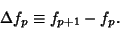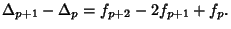## Forward Difference

The forward difference is a Finite Difference defined by(1)

Higher order differences are obtained by repeated operations of the forward difference operator, so(2)

In general,(3)

whereis a Binomial Coefficient.

Newton's Forward Difference Formula expressesas the sum of theth forward differences(4)

whereis the firstth difference computed from the difference table.

See also Backward Difference, Central Difference, Difference Equation, Divided Difference, Reciprocal Difference

References

Abramowitz, M. and Stegun, C. A. (Eds.). Handbook of Mathematical Functions with Formulas, Graphs, and Mathematical Tables, 9th printing. New York: Dover, p. 877, 1972.